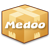version: 2.1.10

### max

Get the maximum value of the column.

max(\$table, \$column, \$where)
• ##### table [string]

The table name.

• ##### column [string]

The target column will be calculated.

• ##### where (optional) [array]

The WHERE clause to filter records.

max(\$table, \$join, \$column, \$where)
• ##### table [string]

The table name.

• ##### join [array]

Table relativity for tables.

• ##### column [string]

The target column will be calculated.

• ##### where (optional) [array]

The WHERE clause to filter records.

Return: [string] The maximum number of the column.
```\$max = \$database->max("account", "age", [
"gender" => "female"
]);

echo "The age of the oldest female user is " . \$max;```